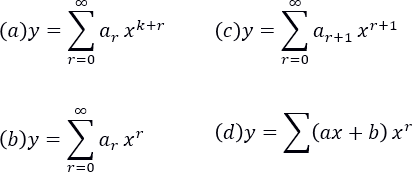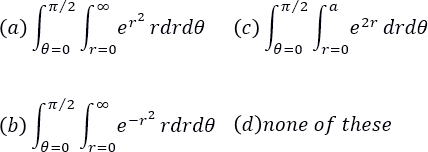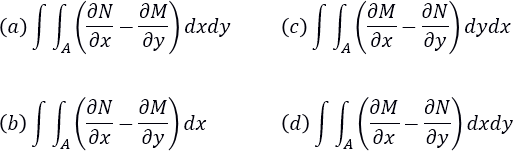MORE IN Engineering Maths 2
VTU First Year Engineering (P Cycle) (Semester 2)
Engineering Maths 2
January 2013
Total marks: --
Total time: --
INSTRUCTIONS
(1) Assume appropriate data and state your reasons
(2) Marks are given to the right of every question
(3) Draw neat diagrams wherever necessary

Choose correct answers for the following:
1 (a) (i) The general solution of the equation p2-5p+6=0 is:
(a) (y-2x-c)(y-3x-c)=0
(b) (y+2x-c)(y+3x-c)=0
(c) (y-2x-c)(y+3x-c)=0
(d) (y-x-c)(y+x-c)=0
1 M
1 (a) (ii) If a differentiable equation is solvable for y then it is of the form:
(a) x=f(y,p)
(b) y=f(x,p)
(c) y=f(x2,py)
(d) x=f(y2,p)
1 M
1 (a) (iii) The differentiable equation of the form y=px+f(p) whose general solution is y=cx+f(c) is known as?
(a) Glairauts equation
(b) Cauchys equation
(c) Lagranges equation
(d) None of these
1 M
1 (a) (iv) The singular solution of the equation y=px-logp is:
(a) y=1-logx
(b) y=1-log(1/x)
(c) y=logx-2x
(d) None of these
1 M
1 (b) Solve the equation p2 + p(x+y) + xy = 0
5 M
1 (c) Solve the equation xp2- 2yp + ax = 0
5 M
1 (d) Obtain the general solution and singular solution of the equation $\sin px \cos y = \cos px \sin y+p$
6 M

Choose correct answers for the following:
2 (a) (i) The homogeneous linear differential equation whose auxiliary equation has roots 1,1,-2 is:
(a) D3 + 3D2 + D + 1 = 0
(b) D3 - 3D + 2 = 0
(c) (D+1)2(D+2) = 0
(d) D3 + 3D + 2 = 0
1 M
2 (a) (ii) The complementary function for the differential equation (D2+2D+1)y = 2x+x2 is:
(a) c1e-x + x2c2e-x
(b) c1ex +c2e-x
(c) (c1+c2)ex
(d) (c1+c2)e-x
1 M
2 (a) (iii) The particular integral of (D2+a2)y = cosax is:
(a) (-x/2a)sinax
(b) (x/2a)cosax
(c) (-x/2a)cosax
(d) (x/2a)sinax
1 M
2 (a) (iv) The general solution of an nth order linear differential equation contains:
(a) at most n constants
(b) exactly n independent constants
(c) atleast n independent constants
(d) more than n constants
1 M
2 (b) Solve: y'' - 2y' + y = xexsinx
5 M
2 (c) Solve: d2y/dx2 - 4dy/dx + 4y = e2x + cosx + 4
6 M
2 (d) Solve: dx/dt = 2x-3y, dy/dt = y-2x given x(0) =8 and y(0) = 3
6 M

Choose correct answers for the following:
3 (a) (i) By the method of variation of parameters,the value of W is called:
(a) Demorgans function
(b) Eulers function
(c) Wronskian function
(d) None of these
1 M
3 (a) (ii) The differential equation of the form a0(ax+b)2y''+a1(ax+b)y'+a2y = φ(x) is called:
(a) Simultaneous equation
(b) Legendres equation
(c) Cauchys equation
(d) Eulers equation
1 M
3 (a) (iii) The equation x3 d3y/dx3 + 3x2 d2y/dx2 + x dy/dx = x3logx by putting x=et with D=d/dt reduces to?
(a) (D3+D2+D)y = 0
(b) D3y = 0
(c) D3y = te3t
(d) none of these
1 M
3 (a) (iv) To find the series solution for the equation 4xd2y/dx2 + 2dy/dx + y = 0,we assume the solution as:1 M
3 (b) Using the variation of parameters method,solve the equation y'' - 2y' + y = ex/x
4 M
3 (c) Solve the equation : x2y'' - xy' + 2y = xsin(logx)
6 M
3 (d) Obtain the Frobenius type series solution of the equation xd2y/dx2 + y = 0
6 M

Choose correct answers for the following:
4 (a) (i) The partial differential equation obtained by eliminating arbitrary constants from the relation Z = (x-a2) + (y-b2) is:
(a) p2 + q2 = 4z
(b) p2 - q2 = 4z
(c) p + q = z
(d) p - q = 2z
1 M
4 (a) (ii) The auxiliary equations of Lagranges linear equation Pp + Qq = R = are:
(a) dx/p = dy/q = dz/R
(b) dx/P = dy/Q = dz/R
(c) dx/x = dy/y = dz/z
(d) dx/x + dy/y - dz/z = 0
1 M
4 (a) (iii) General solution of the equation ∂2z/(∂x∂y) = x2y is:
(a) (1⁄6) x3y2 + f(y) + g(x)
(b) (1⁄6) x2y2 + f(y)
(c) (1⁄6) x3y3
(d) None of these
1 M
4 (a) (iv) By the method of separation of variables,we seek a solution in the form:
(a) X = X(x)Y(y)
(b) Z = X+Y
(c) Z = X2Y2
(d) Z =X/Y
1 M
4 (b) Form a partial differential equation from the relation Z = f(y) + φ(x+y)
5 M
4 (c) Solve the equation (x2 - y2 - z2)p + 2xyq = 2xz
5 M
4 (d) Use the method of separation of variables to solve ∂u/∂x = 2∂u/∂t + u; given that u(x,0) = 6e-3x
6 M

Choose correct answers for the following:
5 (a) (i) 010x2 ey/x dydx is equal to:
(a) 1⁄2
(b) -1⁄2
(c) 1⁄4
(d) 2⁄5
1 M
5 (a) (ii) The integral ∫00e-(x2+y2) dxdy by changing to polar form becomes:1 M
5 (a) (iii) β (3,1⁄2)  is equal to:
(a)  16⁄11
(b)  16⁄15
(c)  15⁄16
(d) 2π/3
1 M
5 (a) (iv) The integral 2∫0e-x2 dx  is:
(a) Γ(3⁄2)
(b) Γ(n+1)
(c) Γ(-1⁄2)
(d) Γ(1⁄2)
1 M
5 (b) Evaluate by changing the order of integration ∫0a02√(xa)x2 dydx; a>0
4 M
5 (c) Evaluate the integral ∫010√(1-x2)0√(1-x2-y2) xyz dzdydx
6 M
5 (d) Prove that ∫0xe-x8dx × ∫0x2e-x4 dx = π/(16√2)
6 M

Choose correct answers for the following:
6 (a) (i) If f = (5xy-6x2)i + (2y-4x)j then ∫Cf·dr where C is the curve y=x3 from the points (1,1) to (2,8) is:
(a) 35
(b) -35
(c) 3x+4y
(d) None of these
1 M
6 (a) (ii) In Green's theorem in the plane ∫C(Mdx+Ndy) = ?1 M
6 (a) (iii) If ∫Cf·dr = 0 then f is called:
(a) Rational
(b) Irrotational
(c) Solenoidal
(d) Rotational
1 M
6 (a) (iv) If all the surfaces are closed in a region containing volume V then the following theorem is applicable:
(a) Stokes theorem
(b) Greens theorem
(c) Gauss divergence theorem
(d) none of these
1 M
6 (b) If f = (2x2-3z)i - 2xyj - 4xk. Evaluate∫v curl f·dv where v is the volume of the region bounded by the planes x=0, y=0, z=0 and 2x+2y+z = 4
4 M
6 (c) Verify Green's theorem for ∫c (3x2 - 8y2)dx + (4y-6xy)dy  where c is the triangle formed by x=0, y=0 and x+y=1
6 M
6 (d) Verify the Stokes theorem for f = -y3 i^+ x3 j^ where s is the circular disc x2 + y2 ≤ 1, z=0
6 M

Choose correct answers for the following:
7 (a) (i) The Laplace transform of f(t)/t when L[ f(t) ] = F(s) is:
(a) ∫0F(s) ds
(b) ∫sF(s) ds
(c) ∫0F(s-a) ds
(d) ∫0F(s+a) ds
1 M
7 (a) (ii) L[t3e2t] = ?
(a) 3!/(s-2)4
(b) 3!/(s+2)4
(c) 3/(s-2)4
(d) 3/(s-2)
1 M
7 (a) (iii) L [f(t-a)H(t-a)] is equal to:
(a) e-asL[f(t)]
(b) easL[f(t)]
(c) e-as/s
(d) L[f(t)]/se-as
1 M
7 (a) (iv) L[δ(t)] is equal to:
(a) 0
(b) -1
(c) e-as
(d) L
1 M
7 (b) Evaluate L[sint sin2t sin3t]
4 M
7 (c) A periodic function of 2π/ω is defined by:
f(t) = Esinωt for 0 ≤ t ≤ π/ω
f(t) = 0  for  π/ω ≤ 1 ≤ 2π/ω
Find  L[f(t)].
6 M
7 (d) Express in terms of unit step function and hence find L[f(t)]
f(t) = 2t , 0 < t ≤ π
f(t) = 1,    t > π
6 M

Choose correct answers for the following:
8 (a) (i) L-1[F(s)/s] is equal to:
(a) ∫01 f(t)·dt
(b) ∫0 f(t)·dt
(c) ∫0 f(t-a)·dt
(d) ∫0t f(t-a)·d
1 M
8 (a) (ii) L-1[1/(s2+2s+5)] is equal to:
(a) etsin2t
(b)  1⁄2e-tsin2t
(c)  1⁄2etcos2t
(d) e2tcos2t
1 M
8 (a) (iii) f(t)×g(t) is defined by:
(a) ∫0t f(t-u)g(u)du
(b) ∫0 f(t)dt
(c) ∫0t f(t)g(t)du
(d) ∫0t f(u)g(u)du
1 M
8 (a) (iv) L-1[1/(s2+a2)] is:
(a) cosat
(b) secat
(c) sinat
(d) (1/a)sinat
1 M
8 (b) Find L-1{(2s-1)/(s2+2s+17)}
4 M
8 (c) By employing the convolution theorem evaluate L-1{s/(s2+a2)2}
6 M
8 (d) Solve the initial value problem y'' - 3y' + 2y = 4t + e3t; y(0)=1, y'(0) = -1 using Laplace transforms
6 M

More question papers from Engineering Maths 2